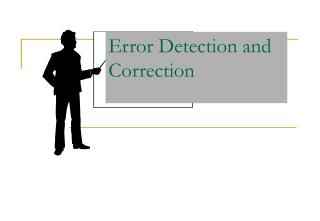DownloadDownload PresentationError Detection and Correction

# Error Detection and Correction

Télécharger la présentation## Error Detection and Correction

- - - - - - - - - - - - - - - - - - - - - - - - - - - E N D - - - - - - - - - - - - - - - - - - - - - - - - - - -
##### Presentation Transcript

1. Error Detection and Correction

2. 9장 Error Detection and Correction 9.1 Types of Errors 9.2 Detection 9.3 Error Correction

3. Error Detection and Correction • Data can be corrupted during transmission. For reliable communication, error must be detected and corrected • are implemented either at the data link layer or the transport layer of the OSI model

4. 9.1 Type of Errors

5. Type of Errors(cont’d) • Single-Bit Error ~ is when only one bit in the data unit has changed (ex : ASCII STX - ASCII LF)

6. Type of Errors(cont’d) • Multiple-Bit Error ~ is when two or more nonconsecutive bits in the data unit have changed(ex : ASCII B - ASCII LF)

7. Type of Errors(cont’d) • Burst Error ~ means that two or more consecutive bits in the data unit have changed

8. 9.2 Detection • Error detection uses the concept of redundancy, which means adding extra bits for detecting errors at the destination

9. Detection(cont’d) • Redundancy

10. Detection(cont’d) • Detection methods • VRC(Vertical Redundancy Check) • LRC(Longitudinal Redundancy) • CRC(Cyclical redundancy Check) • Checksum

11. Detection(cont’d) • VRC(Vertical Redundancy Check) • A parity bit is added to every data unit so that the total number of 1s(including the parity bit) becomes even for even-parity check or odd for odd-parity check • VRC can detect all single-bit errors. It can detect multiple-bit or burst errors only the total number of errors is odd.

12. Detection(cont’d) • Even parity VRC concept

13. Detection(cont’d) • LRC(Longitudinal Redundancy Check) • Parity bits of all the positions are assembled into a new data unit, which is added to the end of the data block

14. VRC and LRC

15. Detection(cont’d) • CRC(Cyclic Redundancy Check) ~ is based on binary division.

16. Detection(cont’d) • CRC generator ~ uses modular-2 division. Binary Division in a CRC Generator

17. Detection(cont’d) Binary Division in a CRC Checker

18. Frame: 1101011011Generator:10011

19. Detection(cont’d) • Polynomials • CRC generator(divisor) is most often represented not as a string of 1s and 0s, but as an algebraic polynomial.

20. Detection(cont’d) • A polynomial representing a divisor

21. Detection(cont’d) • Standard polynomials

22. Detection(cont’d) • Checksum ~ used by the higher layer protocols ~ is based on the concept of redundancy(VRC, LRC, CRC ….)

23. Detection(cont’d) • Checksum Generator

24. Detection(cont’d) • To create the checksum the sender does the following: • The unit is divided into K sections, each of n bits. • Section 1 and 2 are added together using one’s complement. • Section 3 is added to the result of the previous step. • Section 4 is added to the result of the previous step. • The process repeats until section k is added to the result of the previous step. • The final result is complemented to make the checksum.

25. Detection(cont’d) • data unit and checksum

26. Detection(cont’d)

27. 9.3 Error Correction ~ can be handled in two ways  when an error is discovered, the receiver can have the sender retransmit the entire data unit.  a receiver can use an error-correcting code, which automatically corrects certain errors.

28. Error Correction(cont’d) • Single-Bit Error Correction • parity bit • The secret of error correction is to locate the invalid bit or bits • For ASCII code, it needs a three-bit redundancy code(000-111)

29. Error Correction(cont’d) • Redundancy Bits ~ to calculate the number of redundancy bits (R) required to correct a given number of data bit (M)

30. Error Correction(cont’d) • If the total number of bits in a transmittable unit is m+r, then r must be able to indicate at least m+r+1 different states 2r m + r + 1 ex) For value of m is 7(ASCII) , the smallest r value that can satisfy this equation is 4 24 7 + 4 + 1

31. Number of Redundancy Bits (r) Total Bits (m+r) Number of Data Bits (m) 1 2 3 4 5 6 7 2 3 3 3 4 4 4 3 5 6 7 9 10 11 Error Correction(cont’d) • Relationship between data and redundancy bits

32. Error Correction(cont’d) • Hamming Code ~ developed by R.W.Hamming • positions of redundancy bits in Hamming code

33. Error Correction(cont’d) • each r bit is the VRC bit for one combination of data bits r1 = bits 1, 3, 5, 7, 9, 11 r2 = bits 2, 3, 6, 7, 10, 11 r4 = bits 4, 5, 6, 7 r8 = bits 8, 9, 10, 11

34. Error Correction(cont’d) • Redundancy bits calculation(cont’d)

35. Error Correction(cont’d) • Redundancy bits calculation

36. Error Correction(cont’d) • Calculating the r values Calculating Even Parity

37. Error Correction(cont’d) • Error Detection and Correction

38. Error Correction(cont’d) • Error detection using Hamming Code

39. Error Correction(cont’d) • Multiple-Bit Error Correction • redundancy bits calculated on overlapping sets of data units can also be used to correct multiple-bit errors. Ex) to correct double-bit errors, we must take into consideration that two bits can be a combination of any two bits in the entire sequence